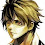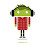# Kotlin 實戰範例 (9) 集合Kotlin 實戰範例

Kotlin 提供的集合處理套件非常好用，而且因為語言本身的設計，在處理集合上更加安全、有效率，我們可以靈活的選擇要建立唯讀的集合，或是不可變動的集合，並且對集合做轉換、過濾、群組、截取、排序及聚合運算等等操作，在某些情況下使用序列運算還可以提升處理效率。

### 9.1 集合概觀

``````fun main() {
// 建立一個陣列
val a = arrayOf("a", "b")
// 加入一個新項目
a.plus("c")
}

// plus() 的原始碼
public actual operator fun <T> Array<T>.plus(element: T): Array<T> {
val index = size
val result = java.util.Arrays.copyOf(this, index + 1)
result[index] = element
return result
}
``````

Kotlin 將集合分成 3 類：

• List：一組有順序、可重覆的集合，可以透過索引值來參照到每個項目的所在位置。`List` 集合和陣列最為相似。
• Set：一組沒有順序、不會重覆的集合，每個項目都是唯一的。
• Map：成對的項目集合，每個項目由唯一鍵 (key) 搭配一個值 (value) 所組成，可稱為鍵值對，值可以重覆，但鍵不能重覆，項目沒有順序的分別。在某些程式語言中被稱為字典 (Dictionary)，因為尋找項目時會比對唯一鍵來找出它的值，就像在查字典一樣。

Kotlin 的標準庫使用泛型來設計處理集合的類別、介面及函式，所以我們可以在任何資料型別上使用相同的集合處理類別、介面及函式。這個集合相關的套件在 kotlin.collections ，內容非常豐富。

#### 9.1.1 集合的類型

Kotlin 的標準庫實作了基本的函式類別，包含 List、Set 及 Map，另外有兩個介面用來表現集合的讀寫能力：

• 可變動的 (mutable)：繼承相對應的唯讀集合，但加上可寫入的功能，例如增加、移除或更新項目。

Kotlin 提供的工具函式可以方便我們快速建立集合，在名稱上使用 `mutable` 開頭的就是可變動的集合，反之就是唯讀類型的集合。在語言的設計上，Kotlin 要求我們明確地使用 `mutable` 名稱來建立可變的集合，確保我們自己知道在做什麼。請看範例：

``````// 宣告唯讀的 List
val a1: List<Int> = listOf<Int>()
// 宣告可變的 List
val a2: MutableList<Int> = mutableListOf<Int>()

// 宣告唯讀的 Set
val b1: Set<String> = setOf<String>()
// 宣告可變的 Set
val b2: MutableSet<String> = mutableSetOf<String>()

// 宣告唯讀的 Map
val c1: Map<Int, String> = mapOf<Int, String>()
// 宣告可變的 Map
val c2: MutableMap<Int, String> = mutableMapOf<Int, String>()
``````

``````// 推薦寫成這樣
val a = listOf<Int>()

// 但是這樣也可以
val b: List<Int> = listOf()

// 如果有初始值則可全部省略
val c = listOf(1, 2, 3)
``````

``````// 唯讀的 List
val a1 = listOf(1, 2, 3)
// 不能做以下的動作
// a1.remove(1)
// a1 = 9

// 可變的 List
val a2 = mutableListOf(1, 2, 3)
// 可以做以下的動作
a2.remove(1) // 刪除
a2 = 9 // 改變值
``````

``````val a11 = a1.toMutableList()

val a22 = a2.toList()
``````

``````val a1 = listOf(1, 2, 3)
println(a1)
// [1, 2, 3]
``````

``````val a = listOf(1, 2, 3)
// a = listOf(4, 5, 6) // 不能對 val 宣告的變數給新值

var b = listOf(1, 2, 3)
b = listOf(4, 5, 6) // 用 var 宣告就可以
``````

``````val c = mutableListOf(1, 2, 3)
c.clear()
``````

#### 9.1.2 List

List 集合的項目是有順序的，可以透過索引值來參照到每個項目的所在位置。索引值從 `0` 開始，最後一個項目的索引值是集合總數減一 `(list.size - 1)` ，我們可以使用 `lastIndex` 屬性比較方便。

Kotlin 將函式提升為和類別同一級，所以不必什麼事都透過類別來做，直接使用函式更有效率，Kotlin 的標準庫中為集合提供了許多便捷的工具函式。Kotlin 針對集合的 getter/setter 做了重載，因此我們可以使用方括號 `[]` 來存取集合的項目，如果要用 `get()``set()` 方法也是可以，但是不推薦。

Array (陣列) 和 List 在各方面都很像，但是有一個重大的差別在於，Array 在宣告時就決定了項目的數量，之後就無法變動；可變動的 List 則沒有這個限制，它可以動態改變項目的數量。Kotlin 對 List 的實作預設為 ArrayList ，我們可以將其視為可以改變容量的陣列。

``````// 唯讀 List
val s1: List<String> = listOf<String>("a", "b", "c")
// 可簡寫如下
val s1 = listOf("a", "b", "c")
// 無法更動項目內容
// s1 = "d"

// 可變動 List
val s2 = mutableListOf("a", "b", "c")
s2 = "d"

// 或是這樣也可以，arrayListOf 回傳 ArrayList
// 而 ArrayList 實作 MutableList
val s3 = arrayListOf("a", "b", "c")
s3 = "d"
``````

``````val s1 = listOf("a", "b", "c")
val s2 = mutableListOf("a", "b")
println(s1 == s2) // false

println(s1 == s2) // true
``````

#### 9.1.3 Set

Set 和 List 的差別在於集合中的項目沒有順序的概念，因此沒有 getter/setter 讓我們透過索引值來存取項目；另外，它的所有項目都是唯一的，也就是不會有任何項目是重覆的，`null` 也只會有一個，請看範例：

``````val a = mutableSetOf<String?>("a", "b", "c")
println(a)
// [a, b, c, d, null]
``````

``````val a = setOf("a", "b", "c")
val b = setOf("c", "a", "b")
println(a == b) // true
``````

MutableSet 是在 Set 之上加入可以修改集合的功能。Kotlin 對 Set 的實作預設為 LinkedHashSet，它實作 `MutableSet` 因此可以修改集合的內容，並且保留了項目插入時的順序，因此當我們呼叫 `frist()``last()` 等等和順序有關的方法時，才能得到預期的項目，請看範例：

``````val a = mutableSetOf("a", "b")
println(a.first()) // a
println(a.last()) // b
println(a.last()) // c
println(a.last()) // d
``````

``````val a = mutableSetOf("a", "b").toHashSet()
// 或
val b = hashSetOf("a", "b")
``````

#### 9.1.4 Map

Map 並沒有實作 `Collection` 介面，但它仍被歸類為集合。Map 儲存的項目為鍵值對 (key-value pairs) ，鍵 (key) 在一個 Map 集合中是唯一的，但是不同的鍵可以有相同的值。

Kotlin 的 Map 項目建立方式和其他程式語言有點不一樣，它使用符號 `to` 來結合鍵和值，請看範例：

``````// 完整寫法
val map: Map<String, Int> = mapOf<String, Int>("a" to 1, "b" to 2, "c" to 3)

// 熟悉以後的寫法
val map = mapOf("a" to 1, "b" to 2, "c" to 3)
println(map.keys) // [a, b, c]
println(map.values) // [1, 2, 3]

// 常用的語法
if ("a" in map) { /* ... */ }
if (map.containsKey("a")) { /* ... */ }
if (map.contains("a")) { /* ... */ }
if (1 in map.values) { /* ... */ }
if (map.containsValue(1)) { /* ... */ }
``````

Map 的等式比較只要鍵值對一樣就會相等，不在乎順序：

``````val map1 = mapOf("a" to 1, "b" to 2, "c" to 3)
val map2 = mapOf("b" to 2, "c" to 3, "a" to 1)
println(map1 == map2) // true
``````

MutableMap 是在 Map 之上加入可以修改項目的功能，來看看怎麼做：

``````val map = mutableMapOf("a" to 1, "b" to 2, "c" to 3)
map.put("a", 5) // 已存在，更新 a 的值
map.put("d", 6) // 不存在，加入新的項目
map["b"] = 7  // 更新 b 的值
println(map)
// {a=5, b=7, c=3, d=6}
``````

Map 使用 `put()` 方法來加入項目，如果 key 相同，資料會被新指定的值覆蓋。Kotlin 也對方括號 `[]` 做了重載，所以我們也可以使用它來讀取或寫入項目。

Kotlin 對 Map 的實作預設為 LinkedHashMap，它會保留項目插入時的順序，如果不需要保留順序，可以改用 HashMap

### 9.2 疊代器

``````val it = listOf(1, 2, 3, 4, 5).iterator()
while (it.hasNext()) {
print(it.next())
}
// 12345
``````

``````val a = listOf(1, 2, 3, 4, 5)
for (it in a) {
print(it)
}
// 12345
``````

Kotlin 的工具函式 `forEach()` 也是使用疊代器，可以將前例改寫如下：

``````fun main() {
// 熟練後的寫法
listOf(1, 2, 3, 4, 5).forEach { print(it) }
// 12345

// 分解動作如下：
// 1.匿名函式
listOf(1, 2, 3, 4, 5).forEach(fun (item: Int) {
print(item)
})
// 2.Lambda 表達式取代匿名函式
listOf(1, 2, 3, 4, 5).forEach( { item -> print(item) } )
// 3.使用預設參數 it
listOf(1, 2, 3, 4, 5).forEach( { print(it) } )
// 4.將 Lambda 移到括號後面並省略括號
listOf(1, 2, 3, 4, 5).forEach { print(it) }
}
``````

`forEach(action: (T) -> Unit)` 只有一個函式型別的參數，也就是要傳入匿名函式，通常使用 Lambda 表達式來簡化；而函式的最後一個參數如果是 Lambda 可以移到括號 `()` 外面並去除括號，因此就成了最終的簡化型式，這個簡化型式在之後會很常用，請務必理解其簡化過程。

### 9.3 範圍

``````val a = 1.rangeTo(5)
println(a)
// 1..5
// 通常會以符號表示
val a1 = 1..5

// for-in 迴圈的應用
for (i in 1..5) {
print(i)
}
// 12345

// 可以轉成 List 來用
(1..5).toList().forEach(::print)
// 12345

// if 條件的應用
val b = 3
if (b in 1..5) {
println("b 在 1~5 之間")
}
// 相當於
if (b >= 1 && b <= 5) {
println("b 在 1~5 之間")
}
``````

``````fun main() {
// 小到大，累進 1
for (i in 1..5) {
print(i)
}
// 12345
// 相當於 Java 中的 for (int i = 1; i <= 5; i++) {}
// Kotlin 沒有 Java 這種型式的迴圈

// 大到小，累進 1
for (i in 5 downTo 1) {
print(i)
}
// 54321
// 也可以寫成 5.downTo(1)

// 小到大，累進 3
for (i in 1..10 step 3) {
print(i)
}
// 14710
// 相當於 Java 的 for (int i = 1; i <= 10; i+=3) {}

// 大到小，累進 3
for (i in 10.downTo(1) step 3) {
print(i)
}
// 10741

// 小到大，不包含尾數
for (i in 1 until 5) {
print(i)
}
// 1234
// 也可以寫成 1.until(5)
// 相當於 Java 的 for (int i = 1; i < 5; i++) {}

// 字元也可以
for (c in 'a'..'e') {
print(c)
}
// abcde
}
``````

### 9.4 集合的運算

• 轉換 (Transformations)：將 A 集合轉換成 B 集合
• 過濾 (Filtering)：或稱篩選，可以挑選或去除符合條件的項目
• 截取集合 (Retrieving collection parts)：取得指定範圍的項目
• 截取項目 (Retrieving single elements)：取得一個指定項目
• 加減運算子 (plus and minus operators)：增加或移除指定項目
• 群組 (Grouping)：將項目分類
• 排序 (Ordering)：將項目依照順序重新排列
• 聚合 (Aggregate operations)：經過運算後得到一個結果值，例如加總

#### 9.4.1 轉換

``````fun main() {
val a = listOf(1, 2, 3)
// 轉成平方值
val b = a.map { it * it }
println(b)
// [1, 4, 9]

// 轉成字串
val c = a.mapIndexed { index, value -> "[\$index] \$value" }
println(c)
// [ 1,  2,  3]

// 奇數值轉成三次方值
// 將偶數設為 null 即可忽略它
val d = a.mapNotNull {
if (it % 2 == 0) null else (it * it * it)
}
println(d)
// [1, 27]
}
``````

#### 9.4.2 過濾

``````fun main() {
/* 過濾 List 集合，也可用於 Set 集合 */
val a = listOf("Tony", "Tom", "Tiffany", "ET", "Jean", "Andy")
// 選擇字母開頭為 T 的名字
val b = a.filter { it.startsWith("T") }
println(b)
// [Tony, Tom, Tiffany]

/* 過濾 Map 集合 */
val c = mapOf(1 to "Tony", 2 to "Tom", 3 to "Tiffany")
// 寫法一：使用預設的參數名稱 it
val d = c.filter { it.key == 1 || it.value.endsWith("y") }
println(d)
// {1=Tony, 3=Tiffany}

// 寫法二：將參數解構為指定名稱
val e = c.filter { (id, name) ->
id == 1 || name.endsWith("y")
}
println(e)
// {1=Tony, 3=Tiffany}
}
``````

#### 9.4.3 群組

``````fun main() {
val a = listOf("Tony", "Tom", "Tiffany", "Andy", "Bob", "Baby")

// 以第一個字母為標準來分類
val b = a.groupBy { it.first() }
println(b)
// {T=[Tony, Tom, Tiffany], A=[Andy], B=[Bob, Baby]}

// 以字串長度為標準來分類
val c = a.groupBy { it.length }
println(c)
// {4=[Tony, Andy, Baby], 3=[Tom, Bob], 7=[Tiffany]}

// 分類的同時對 value 做轉換
val d = a.groupBy ({ it.first() }, { it.toUpperCase() })
println(d)
// {T=[TONY, TOM, TIFFANY], A=[ANDY], B=[BOB, BABY]}
}
``````

#### 9.4.4 截取集合的一部分

``````fun main() {
val a = listOf("0_Tony", "1_Tom", "2_Tiffany", "3_Andy", "4_Bob", "5_Baby")

// 一段範圍
val b = a.slice(2..4)
println(b) // [2_Tiffany, 3_Andy, 4_Bob]

// 偶數索引項目
val c = a.slice(a.indices step 2 )
println(c) // [0_Tony, 2_Tiffany, 4_Bob]

// 以集合指定
val d = a.slice(setOf(4, 1, 3))
println(d) // [4_Bob, 1_Tom, 3_Andy]
}
``````

``````fun main() {
val a = listOf("Tony", "Tom", "Tiffany", "Andy", "Bob", "Baby")

// 拿前 3 個
val b = a.take(3)
println(b) // [Tony, Tom, Tiffany]

// 拿後 2 個
val c = a.takeLast(2)
println(c) // [Bob, Baby]

// 前 3 個丟掉，其他全拿
val d = a.drop(3)
println(d) // [Andy, Bob, Baby]

// 後 2 個丟掉，其他全拿
val e = a.dropLast(2)
println(e) // [Tony, Tom, Tiffany, Andy]

// 前 2 個丟掉，然後拿 2 個
val f = a.drop(2).take(2)
println(f) // [Tiffany, Andy]
}
``````

Tony Blog 撰寫，請勿全文複製，轉載時請註明出處及連結，謝謝 😀

#### 2 則留言

1.您好，我照這上面練習mapIndexed()印出的結果不是[ 1,  2,  3]，我改下發現這樣才印得出來。
原來的:
val c = a.mapIndexed { index, value -> "[index] value" }
println(c) //印出[[index] value, [index] value, [index] value]
我改一下:
val c = a.mapIndexed { index, value -> "[\$index] \$value" }
println(c) //印出[ 1,  2,  3]

1.您好，你的沒錯，是我漏打了 \$ 號，感謝指正 :)

1.回覆時間通常在晚上，如果太忙可能要等幾天。
2.請先瀏覽一下其他人的留言，也許有人問過同樣的問題。
3.程式碼請先將它編碼後再貼上。(線上編碼：http://bit.ly/1DL6yog)
4.文字請加上標點符號及斷行，難以閱讀者恕難回覆。
5.感謝您的留言，您的問題也可能幫助到其他有相同問題的人。